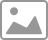搭建 Ulysses 学术写作之公式输入

在 MS Word 输入公式的历史

• 以图片输出，如下图：
• 以 LaTeX 输出，如下图：
• 其实我也不知道 MathML 是什么，也如下图吧：

LaTeX 公式的用法

• 在文章开头加入如下段落：

~~ <script type="text/x-mathjax-config">
~~ MathJax.Hub.Config({tex2jax: {inlineMath:[['$','$']]}});
~~ </script>
~~ <script type="text/javascript" src="http://cdn.mathjax.org/mathjax/latest/MathJax.js?config=TeX-AMS-MML_HTMLorMML"></script>


• 在文中需要输入公式的地方输入

~~$$我是居中公式本人$$
~$我是文中出现的公式$~



	~~E_{s}=\dfrac {E_{i}}{\sqrt {2}}r_{s}e^{-i\left( 2kz_{s}+\varphi \right) } 

完整学术写作的工作流

1. 用 Ulysses 写第一稿，除了排版以外的所有内容；
2. 生成 HTML 文件夹；
3. 全文（纯文本）复制进 MS Word；
4. 用 MathType toggle 公式。

尾巴

Code Matters and Happy Writing!!

74